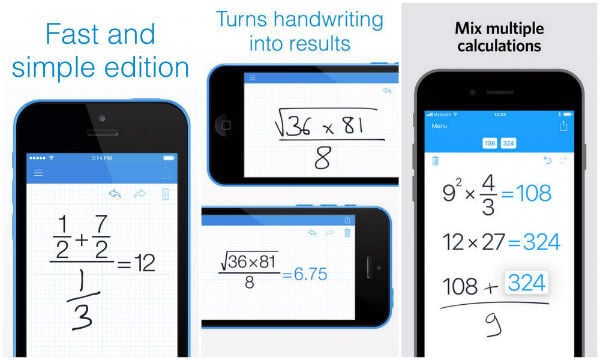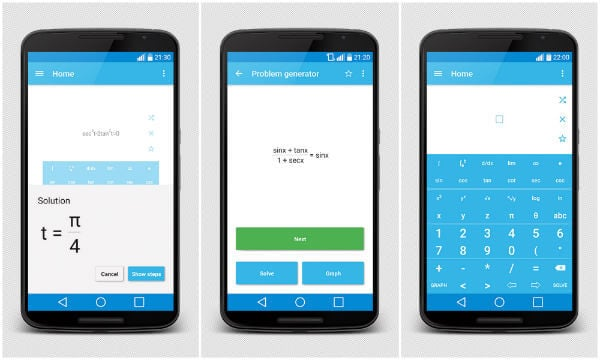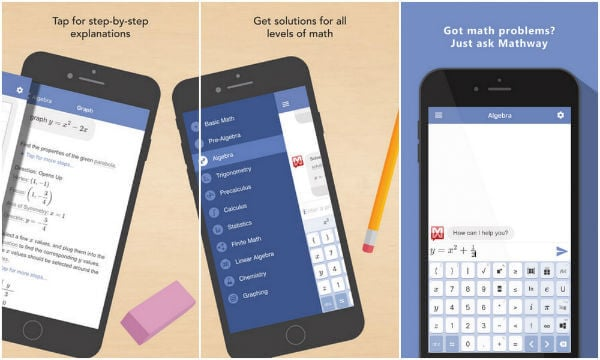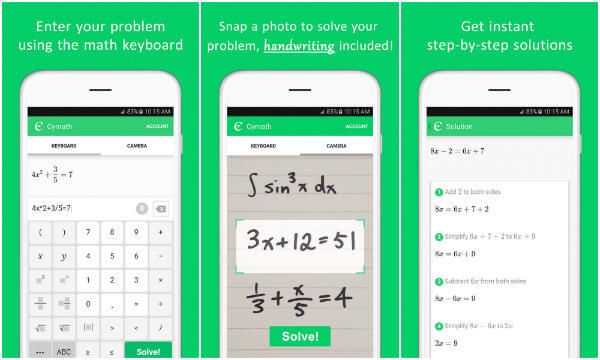HomeMobileAndroid7 Best Math Problem Solver App for Android and iPhone

# 7 Best Math Problem Solver App for Android and iPhone

Math, a tough to crack subject for most of the students, but it is easy to learn if you understand how it works. With the of smartphones, and math solver apps, this is easy now.  This math problem solver apps or camera calculates that bring a solution once you scan the math problem with Android or iPhone camera. This mathematics equation solvers are smart enough to present a step by step solution instead of providing a just final answer. You can easily track diverse formulas and learn the complex algebra in a simple tap on the smartphone.

Whether it is arithmetic, calculus or algebra, these math problem solver apps are available for both Android and iOS are ready to explain math problems with step by step explanations.

### Photomath

Photomath is a math solver for Android and iPhone app that simply works just by placing the smartphone over the math problem. It uses your smartphone’s camera for analyzing the problem and giving the solution instantaneously. The result is given out in a detailed step-by-step instructional manner with a smart calculator. You can easily solve the problem by writing it on the app’s screen.The user can easily solve the mathematical problems based on integers, arithmetic, decimal, algebraic expressions, graphs and much more. This app is providing animated explanations and the smartphone user just needs to copy down the solution from the device to their notebook. This math problem solver app supports about 36 languages at all to help a large number of people.

### MyScript Calculator

Get math problems solution in real time with the MyScript Calculator. The math solver app from MyScript Interactive Ink that was present in the previous edition. This math app allows the user to hand write the math problem on the app to get better solutions. With scratching out gestures, you can easily erase the unwanted area. MyScript gives the feeling of writing on the paper and solving it over the app.We can either choose to solve the problem on the subsequent line or do several calculations on more than one line. Copy the solution on the clipboard and export it to other apps. You can always reuse the results anytime in future. Retrieve all your previous calculations whenever you need them. In addition to this, you can redo and undo the calculations made on the app.

### MalMath

Understand the solving process with great depth through MalMath. This math equation solver is highly helpful for the high school as well as college students. The app acquires the highlighted option making the students easier to understand steps. You can easily exercise their brain with the math problems available in several categories and levels of difficulty.After solving the math problems, you can easily share or save them for future access. With graph analysis feature on the app, get instant solutions to maths problems based on graphs. The math solver app is free to use and solves logarithms, equations, algebra and much more with utmost simplicity. You can easily customize this math solver app to use in your language to understand the solution in a better way.

### Mathway

Receive instant answers to your troubling math sums with this Mathway App. The app is easy to use and efficient in every manner. Mathway comes with an appealing interface for most of the people who are in dire need of solutions. You will get to know the math solving tips to reach the answer and learn that process to solve it again on your own. With this camera calculator, you can simply input the problem or capture it via device’s camera to get the solution.This math question solver offers an experience of working with private mathematics tutor. Got any tough math problem, then Mathway is here to help. For step-by-step solutions, the person needs to buy the monthly or annual subscription.

### Wolfram Alpha

Wolfram Alpha brings great knowledge and the deep power of computation aiding the users to solve their math problems. Whether the math problem is of arithmetic, calculus or etc., this math equation solver app is always ready for the challenge. You can access the maths app for solving the homework and get on the spot solution of any problem.iOS or Android users can download and install the app for ascertaining the means to avoid the mental maths trouble. Use this app for more than just solving the math problems whenever you feel the need to do so. The computational knowledge engine of the app covers a good number of subjects and topics serving as an instant knowledge expert to you.

### Socratic

For a large number of people, Socratic is a great homework helper assisting in every process of getting the solutions to questions. The math solver app works as soon as you grab the photo of your maths question. This math solution app helps the user to learn the concepts by providing the instant explanations, tutorials, and step-by-step instruction set.The maths app’s AI accurately predicts the concept that will surely assist you in solving the question. The user interface of the math solver for Android inherits innovative technology that read the question present on the image and gives the solution immediately. Learn better and faster in a way like never before with the visual content offered by the developers in different subjects. Watch the online videos on the app that are shortlisted from sources like Khan Academy, Crash Course, and similar to understand any topic or subject better

### Cymath

Cymath app uses the same math engine present on its official website. You can solve your math problems on the go based on a wide variety of topics. Access step-by-step solutions to your problems without any cost on this math solver app. Stuck on any math problem or require specific calculus help, then use this Cymath app.Let the app to be your regular partner in your maths homework. Allow the math solver app to give you the necessary aid regarding the maths homework whether it is based on algebra, calculus and graphing. For additional assistance, the iOS/Android user can opt for Cymath Plus available at \$4.99 USD per month.

## Math Solver App for Android iPhone

There is no denying on the fact that mathematics is a really hard subject. Most of the adults do remember how the subject victimized them in their childhood. Back in the old days, there were good solvers present in the market. These solvers worked as an aid to provide solutions to each and every mathematical problem. However, these books were quite bulky and students of the current generation need something that is ultra portable. Now, they can bid adieu to these books, just by using the math solver apps available for Android and iOS.

These math problem-solving apps aids in giving you the solution of the problem fast with step by step explanations. Know whether you are following the right way to solve the equations with these maths app and get best results. Math problem solver apps are a lifesaver for students, who did not understood the concept fully, but have to submit the homework on next day. Use the math question solver app’s camera calculator feature to state the problem that is avoiding the need to write it entirely. Solve any sort of maths problems with these math question solver apps.

Disclosure: Mashtips is supported by its audience. As an Amazon Associate I earn from qualifying purchases.

1. Try this app called ThirdLeap Math. It acts like a personal pocket math guide which challenges you to learn to think. I’ve recently been using this to brush my math and trust me its super eazy !!!!

2.Webby Giants

Solve4x is another best free option for iOS user. In this app, you can simply enter the equation or question manually and get the solution or you can take the image which will automatically solve the entire question or equation.

3.Pasquale Capozzola